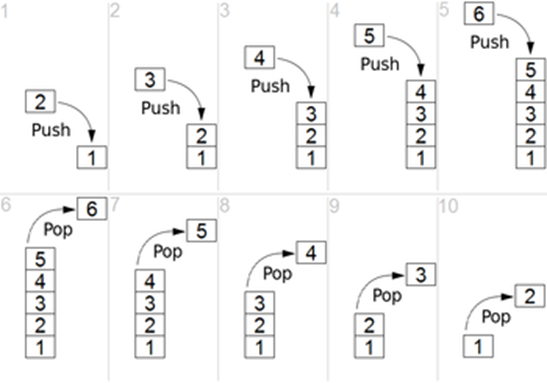# Stack vs Heap – Difference between Stack and Heap in Data Structure

In this tutorial you will learn about stack vs heap data structures.

Stack

Stack is a simple data structure used for storing data. In stack, the order in which the data arrives is important. A suitable example for stack is, a pile of plates in kitchen. When we want a plate we will take which was last placed in that pile. This is the main property of stack we say Last in first Out (LIFO) or First in Last out (FILO). So stack is a ordered list in which insertion and deletion are done at one end, called top.

Stack Operations:

Push: When an element inserted into the stack, we call it as push operation.

Top: When we want to know what is the top element of the stack we get it by top operation.

Pop: When we remove an element from the top of the stack, we call it as pop operation.

IsEmptyStack(): To check whether stack is empty or not.

IsFullStack(): To check stack is full or not.

And some other properties are,

Stack underflow: When we want to pop an element from the empty stack, that situation is called stack underflow.

Stack overflow: When we want to push an element to a full stack (i.e stack elements equal to stack size), that situation is called stack overflow.Also Read: Difference Between Stack and Queue

Heap

Heap is a tree with some special property. That special property of the heap is, the value of a node must be >= or <= to its children. And one most important property of heap is all leaves must be at level h or at h-1. (where h is the height of the tree). This also called heap must be a complete binary tree.

Types of Heaps:

Min Heap: The value of the node must be less than or equal to the values of its children.

Max Heap: The value of the node must be greater than or equal to the values of its children.

Finding minimum element, deleting minimum element, are easy operations in min heap.

Finding maximum element, deleting maximum element, are easy operations in max heap.Binary Min HeapBinary Max Heap

## Stack vs Heap – Difference between Stack and Heap

 Stack Heap 1) Stack is a linear data structure. 1) Heap is a hierarchical data structure. 2) Elements in stack follow LIFO or FILO property. 2) Elements in heap follow heap property (mentioned above). 3) Push, Pop, Top are the only allowed operations on the stack. 3) Almost all tree operations can be applied on heaps. Heapifying an element, find minimum, find maximum are some examples. 4) All mentioned operations, push, pop, top, IsEmptyStack(), IsFullStack(), Size(), takes O(1) time in stack. 4) In heap, insertion takes O(log n) time. And finding min, deleting min (in min – heap), finding max, deleting max (in max – heap) takes O(1) time. 5) Stack can be implemented in 3 ways that, simple array based, dynamic array based and Linked list based implementation. 5) Heap can be implemented using arrays, and trees. 6) We can limit stack size, so that stack overflow, stack underflow conditions will occur. 6) There is no case of overflow and underflow in heap. 7) Balancing of symbols, infix – to – postfix conversions, undo sequence in a text editor, implementing function calls (especially recursion) are some applications of stacks. 7) Heaps can be useful for heapsort (best performance), selection algorithms (finding min, finding max, finding median, kth largest/smallest, etc), Graph algorithms.

Comment below if you have queries related to above tutorial for stack vs heap.

### 1 thought on “Stack vs Heap – Difference between Stack and Heap in Data Structure”

1.write a program that reads a string from a text file. reverse the string using the stack and write the reversed string to another text file